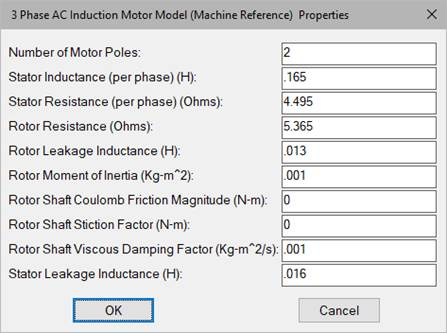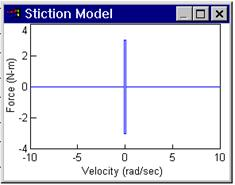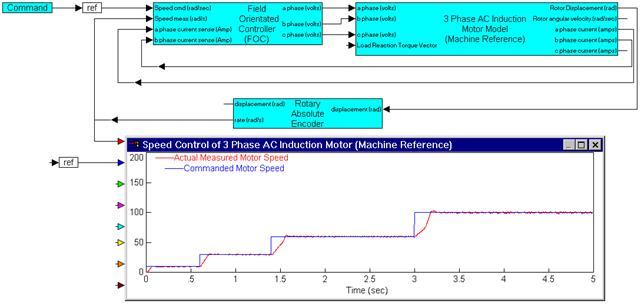##### PWM Brush Amplifier (2Q, Current Feedback)Category: eMotors > Controllers > PWM Control

The PWM Brush Motor Amplifier (2Q, Current Feedback) <tbs, will Mayank add Amplifier to the block name> block simulates a brush DC motor unipolar PWM amplifier (2 quadrant) with current feedback that can be used for unidirectional motor control. The control logic simulates a two-device inverter that allows PWM swing from 0 to the specified supply voltage over a 0 to 100% modulation range. An inhibit control is provided to disable amplifier output for a control voltage less than 0.3 V (logic low). Control of the PWM is accomplished through current feedback control. Motor current is fed back through a current sense (resistor). The controller for the current loop is PI; the integral and proportional gains can be specified by you. By selecting appropriate values of PI proportional and integral gain, current loop dynamics can be adjusted for any motor. Logic is provided that clamps the output to limit load current according to the specified current limit. Anti-windup control is provided in the integrator.

Motor (+) output provides the PWM control signal; Motor (-) is referenced at a constant 0 V.

To provide adequate simulation of PWM behavior, the simulation step size should be considerably smaller than the inverse of the selected PWM frequency; preferably at least 100 times smaller.

Direct access to the PWM can be accomplished by setting the current sense gain and current loop integral gain equal to 0, and the current loop proportional gain equal to 1. In this situation, current limiting is still available as long as motor current output is connected to the current sense input.Supply Voltage: sets the voltage level output of the PWM in volts.

PWM Frequency: sets the carrier frequency for the PWM in hertz. Note that to provide adequate simulation of PWM behavior, the simulation step size should be chosen to be considerably smaller than the inverse of the selected PWM frequency; preferably at least 100 times smaller.

Current Loop Integral Gain: Typically sets the speed and tracking accuracy (bandwidth) of the current control loop. Units are 1/sec.

Current Loop Proportional Gain: Typically sets the damping of the current control loop.

Current Sense Gain: Sets the gain of the current feedback-sensing device. Units are in amps/amp.

Input: The amplifier input must be a positive voltage. The amplifier saturates beyond the range of 0 to 100% modulation. To avoid operation beyond saturation the input should be limited between 0 V and the supply voltage divided by the amplifier gain.

Current Limit: Sets the maximum average output current the amplifier will provide. Units are in amps.

Inhibit: Provides immediate inhibiting of the output signal and can be used with limit switches for out-of-range control. Active when input is less than 0.3 V (active low).

#### Example

Diagram name: PWM Brush Amplifier 2Q WCF <tbs, will Mayank change name to Current Feedback>

Location: Examples > eMotor > DC Brush

This example illustrates how the PWM amplifier can be used to directly regulate a set current in a DC motor. Such a control loop may be used to control against torque disturbance since torque is directly proportional to motor current. Here the supply voltage is set at 180 V, and PWM frequency is 9000 Hz. Proportional gain is set at 0, so the control is pure integral with a gain of 50. The current sense gain is 1, so the average controlled current should approach the set current of 1.5 amps.